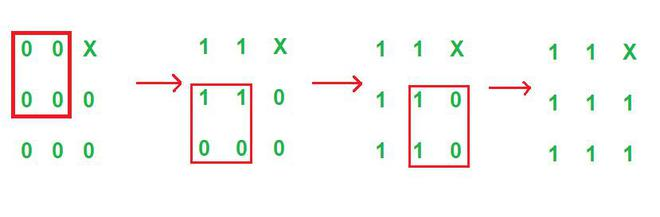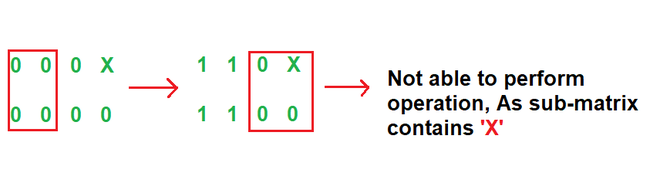GFG App
Open AppBrowser
Continue

# Check if it is possible to make all elements into 1 except obstacles

Given a matrix M[][] of size N * M containing characters 0 and X. You have to choose a sub-matrix of 2*2 size, which doesn’t contains X in it, and convert all the 0 under that sub-matrix into 1. Update M[][] after each operation. Return “YES“, otherwise “NO” If it is possible to make all the zeros into ones except the cell containing X by using the given operation.

Examples:

Input: N = 3, M = 3

M[][]:

0 0 X
0 0 0
0 0 0
Output: YES
Explanation:Graphical Explanation of input test case 1

It can be seen that from the initial matrix, at each operation a sub-matrix of size 2*2 is chose and convert all the zeros into ones under those sub-matrices using the given operation. It also noted that some sub-matrices contain 1 also, in this case remain the same. Any sub-matrix of size 2*2 doesn’t contains ‘X’. Therefore, it is possible to convert all the zeros into ones using given operation except X. Hence output is YES.

Input: N = 4, M = 2

M[][]:

0 0 0 X
0 0 0 0
Output: NO
Explanation:Graphical explanation of input test case 2

It can be verified that all zeros in initial matrix can’t be converted into ones.

Approach: Implement the idea below to solve the problem:

The problem is observation based and can be solved by using those observations.

Steps were taken to solve the problem:

1. Create a StringBuilder Array let’s say X[] of size N.
2. Initialize X[] with input matrix M[][].
3. Run two nested loops for i = 0 to i < N – 1 and j = 0 to j < M – 1 and follow the below-mentioned steps under the scope of the loop:
• If ( X[ i ].charAt( j ) == ‘0’ || X[ i ].charAt( j ) == ‘1’ )
• If ( X[ i ].charAt(j + 1) != 88 && X[i + 1].charAt(j + 1) != 88 && X[i + 1].charAt( j ) != 88)
• X[ i ].setCharAt(j, ‘1’)
• X[ i ].setCharAt(j + 1, ‘1’)
• X[i + 1].setCharAt(j, ‘1’)
• X[i + 1].setCharAt(j + 1, ‘1’)
4. Now, just traverse the X[] and check if there exists 0 or not. If 0 exists then output NO or else YES

Below is the code to implement the approach:

## C++

 `// C++ code to implement the approach`   `#include ` `using` `namespace` `std;`   `// Function for checking is it possible` `// to make all zeros into ones` `void` `Is_Possible(``int` `N, ``int` `M, vector & x)` `{`   `    ``// Implementing the approach on original matrix` `    ``for` `(``int` `i = 0; i < N - 1; i++) {`   `        ``for` `(``int` `j = 0; j < M - 1; j++) ` `        ``{` `            ``if` `(x[i][j] == ``'0'` `|| x[i][j] == ``'1'``) ` `            ``{` `                ``if` `(x[i][j + 1] != ``'X'` `&& ` `                    ``x[i + 1][j + 1] != ``'X'` `&&` `                    ``x[i + 1][j] != ``'X'``) ` `                ``{` `                    ``x[i][j] = ``'1'``;` `                    ``x[i][j + 1] = ``'1'``;` `                    ``x[i + 1][j] = ``'1'``;` `                    ``x[i + 1][j + 1] = ``'1'``;` `                ``}` `            ``}` `        ``}` `    ``}`   `    ``// Flag initialized and set to false` `    ``bool` `flag = ``false``;`   `    ``// Traversing vector of string x[]` `    ``// to check if there is an element` `    ``// exists any 0 Which is still not` `    ``// converted into 1` `    ``for` `(``int` `i = 0; i < N; i++) {`   `        ``for` `(``int` `j = 0; j < M; j++) {` `            ``if` `(x[i][j] == ``'0'``) {` `                ``cout << ``"NO"` `<< endl;` `                ``flag = ``true``;` `                ``break``;` `            ``}` `        ``}` `        ``if` `(flag)` `            ``break``;` `    ``}` `    ``if` `(!flag)` `        ``cout << ``"YES"` `<< endl;` `}`   `int` `main() {`   `       ``// Inputs` `       ``int` `N = 4;` `       ``int` `M = 2;` `       ``vector arr = { ``"00"``, ``"00"``, ``"00"``, ``"0X"` `};`   `    ``// Function call` `    ``Is_Possible(N, M, arr);` `}`

## Java

 `// Java code to implement the approahc` `import` `java.util.*;`   `class` `GFG {`   `    ``// Driver Function` `    ``public` `static` `void` `main(String az[])` `    ``{`   `        ``// Inputs` `        ``int` `N = ``4``;` `        ``int` `M = ``2``;` `        ``String[] arr = { ``"00"``, ``"00"``, ``"00"``, ``"0X"` `};`   `        ``// Function call` `        ``Is_Possible(N, M, arr);` `    ``}`   `    ``// Function for checking is it possible` `    ``// to make all zeros into ones` `    ``static` `void` `Is_Possible(``int` `N, ``int` `M, String[] arr)` `    ``{`   `        ``// StringBuilder array object is` `        ``// initialized to hold matrix` `        ``StringBuilder x[] = ``new` `StringBuilder[N];`   `        ``// Loop for initializing` `        ``// StringBuilder array` `        ``for` `(``int` `i = ``0``; i < N; i++) {`   `            ``x[i] = ``new` `StringBuilder(arr[i]);` `        ``}`   `        ``// Implementing the approach` `        ``for` `(``int` `i = ``0``; i < N - ``1``; i++) {`   `            ``for` `(``int` `j = ``0``; j < M - ``1``; j++) {` `                ``if` `(x[i].charAt(j) == ``'0'` `                    ``|| x[i].charAt(j) == ``'1'``) {` `                    ``if` `(x[i].charAt(j + ``1``) != ``88` `                        ``&& x[i + ``1``].charAt(j + ``1``) != ``88` `                        ``&& x[i + ``1``].charAt(j) != ``88``) {` `                        ``x[i].setCharAt(j, ``'1'``);` `                        ``x[i].setCharAt(j + ``1``, ``'1'``);` `                        ``x[i + ``1``].setCharAt(j, ``'1'``);` `                        ``x[i + ``1``].setCharAt(j + ``1``, ``'1'``);` `                    ``}` `                ``}` `            ``}` `        ``}`   `        ``// Below lines will print` `        ``// StringBuilder object(Should be` `        ``// use only for debugging)`   `        ``// for(StringBuilder X : x)` `        ``// System.out.println(X);`   `        ``// Flag initialized and set to false` `        ``boolean` `flag = ``false``;`   `        ``// Traversing StringBuilder array` `        ``// to check if there is an element` `        ``// exists any 0 Which is still not` `        ``// converted into 1` `        ``for` `(``int` `i = ``0``; i < N; i++) {`   `            ``for` `(``int` `j = ``0``; j < M; j++) {` `                ``if` `(x[i].charAt(j) == ``'0'``) {` `                    ``System.out.println(``"NO"``);` `                    ``flag = ``true``;` `                    ``break``;` `                ``}` `            ``}` `            ``if` `(flag)` `                ``break``;` `        ``}` `        ``if` `(!flag)` `            ``System.out.println(``"YES"``);` `    ``}` `}`

## C#

 `// C# code to implement the approahc` `using` `System;` `using` `System.Text;`   `public` `class` `GFG{`   `    ``// Function for checking is it possible` `    ``// to make all zeros into ones` `    ``static` `void` `Is_Possible(``int` `N, ``int` `M, ``string``[] arr)` `    ``{` `    ``// StringBuilder array object is` `        ``// initialized to hold matrix` `        ``StringBuilder[] x = ``new` `StringBuilder[N];`   `        ``// Loop for initializing` `        ``// StringBuilder array` `        ``for` `(``int` `i = 0; i < N; i++) {`   `            ``x[i] = ``new` `StringBuilder(arr[i]);` `        ``}`   `       ``for` `(``int` `i = 0; i < N - 1; i++) {`   `            ``for` `(``int` `j = 0; j < M - 1; j++) ` `            ``{` `                ``if` `(x[i][j] == ``'0'` `|| x[i][j] == ``'1'``) ` `                ``{` `                    ``if` `(x[i][j + 1] != ``'X'` `&& ` `                        ``x[i + 1][j + 1] != ``'X'` `&&` `                        ``x[i + 1][j] != ``'X'``) ` `                    ``{` `                        ``x[i][j] = ``'1'``;` `                        ``x[i][j + 1] = ``'1'``;` `                        ``x[i + 1][j] = ``'1'``;` `                        ``x[i + 1][j + 1] = ``'1'``;` `                    ``}` `                ``}` `            ``}` `        ``}`   `     `  `        ``// Flag initialized and set to false` `        ``bool` `flag = ``false``;`   `        ``// Traversing StringBuilder array` `        ``// to check if there is an element` `        ``// exists any 0 Which is still not` `        ``// converted into 1` `        ``for` `(``int` `i = 0; i < N; i++) {`   `            ``for` `(``int` `j = 0; j < M; j++) {` `                 ``if` `(x[i][j] == ``'0'``) {` `                    ``Console.WriteLine(``"NO"``);` `                    ``flag = ``true``;` `                    ``break``;` `                ``}` `            ``}` `            ``if` `(flag)` `                ``break``;` `        ``}` `        ``if` `(!flag)` `            ``Console.WriteLine(``"YES"``);` `    ``}` `    ``static` `public` `void` `Main (){`   `         ``// Inputs` `        ``int` `N = 4;` `        ``int` `M = 2;` `        ``string``[] arr = { ``"00"``, ``"00"``, ``"00"``, ``"0X"` `};`   `        ``// Function call` `        ``Is_Possible(N, M, arr);` `    ``}` `}`

## Python

 `# Function for checking if it is possible to make all zeros into ones` `def` `is_possible(n, m, arr):`   `    ``# Initialize a list of lists to hold the matrix` `    ``x ``=` `[``list``(row) ``for` `row ``in` `arr]`   `    ``# Implementing the approach` `    ``for` `i ``in` `range``(n ``-` `1``):`   `        ``for` `j ``in` `range``(m ``-` `1``):` `            ``if` `x[i][j] ``=``=` `'0'` `or` `x[i][j] ``=``=` `'1'``:` `                ``if` `x[i][j ``+` `1``] !``=` `'X'` `and` `x[i ``+` `1``][j ``+` `1``] !``=` `'X'` `and` `x[i ``+` `1``][j] !``=` `'X'``:` `                    ``x[i][j] ``=` `'1'` `                    ``x[i][j ``+` `1``] ``=` `'1'` `                    ``x[i ``+` `1``][j] ``=` `'1'` `                    ``x[i ``+` `1``][j ``+` `1``] ``=` `'1'`   `    ``# Traversing list of lists to check if there is any 0 which is still not converted into 1` `    ``for` `i ``in` `range``(n):`   `        ``for` `j ``in` `range``(m):` `            ``if` `x[i][j] ``=``=` `'0'``:` `                ``return` `"NO"`   `    ``return` `"YES"`     `# Driver code` `if` `__name__ ``=``=` `"__main__"``:`   `    ``# Inputs` `    ``n ``=` `4` `    ``m ``=` `2` `    ``arr ``=` `[``"00"``, ``"00"``, ``"00"``, ``"0X"``]`   `    ``# Function call` `    ``print``(is_possible(n, m, arr))`

## Javascript

 `// JavaScript code to implement the approach`   `// Function for checking is it possible` `// to make all zeros into ones` `function` `Is_Possible(N, M, arr) {` `    ``// Implementing the approach on original matrix` `    ``for` `(let i = 0; i < N - 1; i++) {`   `        ``for` `(let j = 0; j < M - 1; j++) {` `            ``if` `(arr[i][j] == ``'0'` `|| arr[i][j] == ``'1'``) {` `                ``if` `(arr[i][j + 1] != ``'X'` `&&` `                    ``arr[i + 1][j + 1] != ``'X'` `&&` `                    ``arr[i + 1][j] != ``'X'``) {` `                    ``arr[i] = arr[i].substr(0, j) + ``'11'` `+ arr[i].substr(j + 2, M);` `                    ``arr[i + 1] = arr[i + 1].substr(0, j) + ``'11'` `+ arr[i + 1].substr(j + 2, M);` `                ``}` `            ``}` `        ``}` `    ``}`   `    ``// Flag initialized and set to false` `    ``let flag = ``false``;`   `    ``// Traversing array of strings arr[]` `    ``// to check if there is an element` `    ``// exists any 0 Which is still not` `    ``// converted into 1` `    ``for` `(let i = 0; i < N; i++) {`   `        ``for` `(let j = 0; j < M; j++) {` `            ``if` `(arr[i][j] == ``'0'``) {` `                ``console.log(``"NO"``);` `                ``flag = ``true``;` `                ``break``;` `            ``}` `        ``}` `        ``if` `(flag)` `            ``break``;` `    ``}` `    ``if` `(!flag)` `        ``console.log(``"YES"``);` `}`   `// Inputs` `let N = 4;` `let M = 2;` `let arr = [``"00"``, ``"00"``, ``"00"``, ``"0X"``];`   `// Function call` `Is_Possible(N, M, arr);`

Output

`NO`

Time Complexity: O(N*M)
Auxiliary Space: O(N*M), As StringBuilder is used of size N*M.

My Personal Notes arrow_drop_up